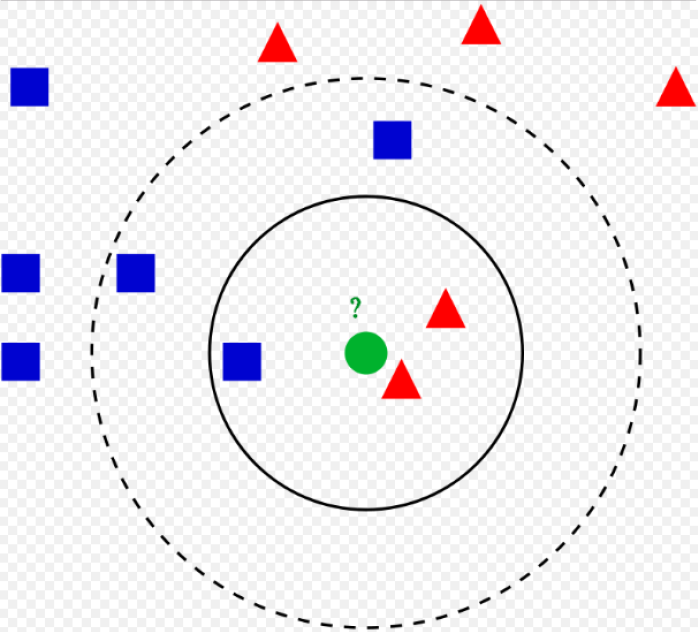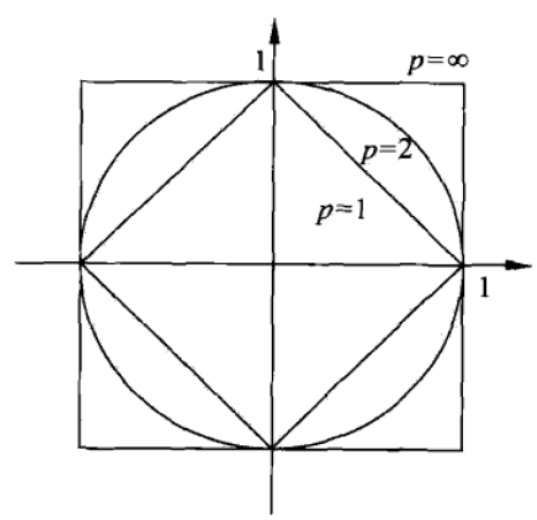# 1 算法原理

## 1.1 $k$近邻算法

**输入：**训练数据集$T=\left[\left(x_{1}, y_{1}\right), \cdots,\left(x_{N}, y_{N}\right)\right), x_{i} \in X \subseteq R^{n}, y_{i} \in Y=\left\{c_{1}, \cdots, c_{K}\right\}$，实例特征向量$x$

1. 根据给定的距离度量（欧氏距离），在训练集中找到与$x$最近的$k$个点，包括这$k$个点的区域记为$N_{k}(x)$
2. $N_{k}(x)$中根据分类决策规则（如多数表决）决定$x$的类别$y$.图1.KNN算法

## 1.2 $k$近邻模型

### 1.2.1 模型

$k$近邻方法没有显式的模型。

### 1.2.2 距离度量

$L_{p}$距离（明可夫斯基距离）
$p$范数$\left\|x-x_{i}\right\|_{p}$来度量，$L_{p}$距离定义如下：
$L_{p}\left(x_{i}, x_{j}\right)=\left(\sum_{l=1}^{n}\left|x_{i}^{(l)}-x_{j}^{(l)}\right|^{p}\right)^{\frac{1}{p}}$1. 曼哈顿距离：当$p=1$时，为曼哈顿距离，如图中，在小正方形上的各点距离原点的曼哈顿距离相等。
2. 欧式距离：当$p=2$时，为欧氏距离，如图，在圆上的各点与原点的欧氏距离相等。
3. 切比雪夫距离：当$p=\infty$时，为切比雪夫距离。对应大正方形上各点到原点的切比雪夫距离相等。

### 1.2.3 $k$值的选择

$k$值的选择会对$k$近邻算法的结果产生重大的影响。$k$值的减小，会使预测结果非常依赖近邻点，如近邻点刚好是噪声，预测会出错。$k$值的减小意味着模型变得复杂，容易发生过拟合。

### 1.2.4 分类决策规则

$\frac{1}{k} \sum_{x_{i} \in N_{k}(x)} I\left(y_{i} \neq c_{j}\right)$

## 1.3总结

1. 第2章的感知机模型，需要使用所有的训练集数据，找到一个可以分割两类的超平面，并要求数据是线性可分的，当然对于数据不是线性可分时，也有相应的感知机算法。当找到这个超平面，训练数据集就可以不再需要了，仅根据该超平面，就可以判定新实例的类别。总的来说，感知机模型用全部数据找到了一个全局的结构，保留这个结构并遗弃训练集数据，用该结构预测新实例。
2. 第3章的近邻算法，并没有用全部数据寻找一个结构，所用的是一个局部的信息，所找的是要预测的这个实例离该点最近的那些点，然后使用这些实例进行预测。当使用局部信息时，需要存储全部的训练数据集。要如何在这两种算法中选择呢？当数据具有线性可分结构时，用感知机模型更好，因为计算简单，但当数据不是线性可分时，只能使用预测点周围的信息（局部信息）进行分类判定，此时近邻算法更好，该算法对整个数据集的结构（即模型的结构）没有那么强的假设。

# 2 算法实现

©️2019 CSDN 皮肤主题: 大白 设计师: CSDN官方博客